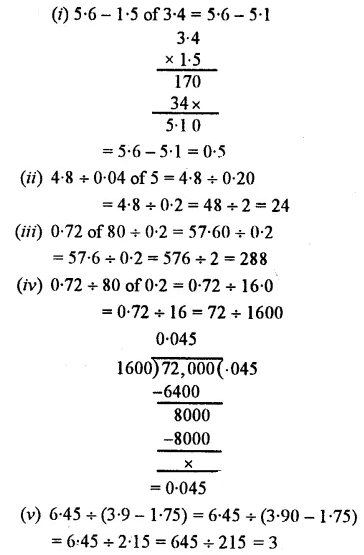# Selina Concise Mathematics Class 7 ICSE Solutions Chapter 4 Decimal Fractions

## Selina Concise Mathematics Class 7 ICSE Solutions Chapter 4 Decimal Fractions (Decimals)

Selina Publishers Concise Mathematics Class 7 ICSE Solutions Chapter 4 Decimal Fractions (Decimals)

### Decimal Fractions Exercise 4A – Selina Concise Mathematics Class 7 ICSE Solutions

Question 1.
Convert the following into fractions in their lowest terms :
(i) 3.75
(ii) 0.5
(iii) 2.04
(iv) 0.65
(v) 2.405
(vi) 0.085
(vii) 8.025
Solution: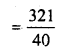Question 2.
Convert into decimal fractions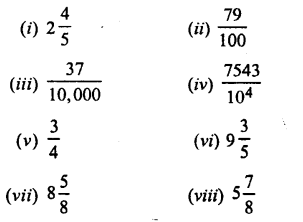Solution:Question 3.
Write the number of decimal places in :
(i) 0.4762
(ii) 7.00349
(iii) 8235.403
(iv) 35.4
(v) 2.608
(vi) 0.000879
Solution: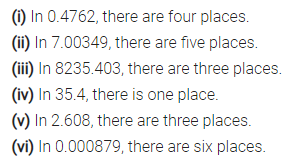Question 4.
Write the following decimals as word statements :
(i) 0.4,0.9,0.1
(ii) 1.9, 4.4, 7.5
(iii) 0.02, 0.56, 13.06
(iv) 0.005,0.207, 111.519
(v) 0.8, 0.08, 0.008, 0.0008
(vi)256.1, 10.22, 0.634
Solution: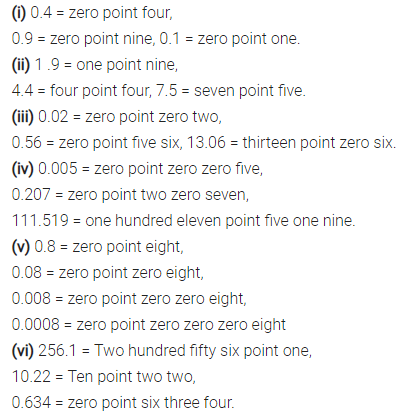Question 5.
Convert the given fractions into like fractions:
(i) 0.5,3.62,43.987 and 232.0037
(ii) 215.78, 33.0006, 530.3 and 0.03569
Solution: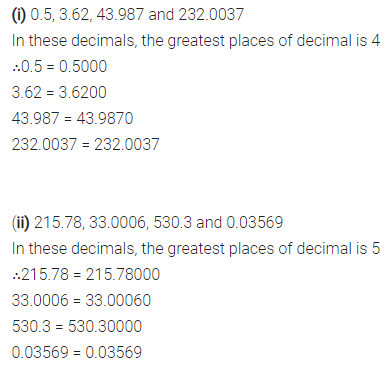### Decimal Fractions Exercise 4B – Selina Concise Mathematics Class 7 ICSE Solutions

Question 1.
(i) 0.5 and 0.37 (ii) 3.8 and 8.7
(iii) 0.02, 0.008 and 0.309
(iv) 0. 4136, 0. 3195 and 0.52
(v) 9.25, 3.4 and 6.666
(vi) 3.007, 0.587 and 18.341
(vii) 0.2, 0.02 and 2.0002
(viii) 6. 08, 60.8, 0.608 and 0.0608
(ix) 29.03, 0.0003, 0.3 and 7.2
(x) 3.4, 2.025, 9.36 and 3.6221
Solution: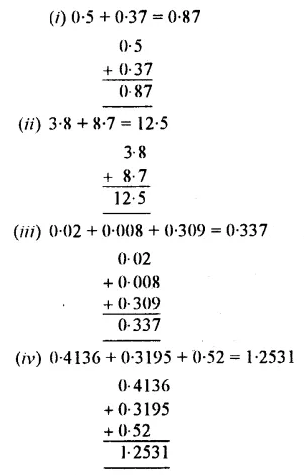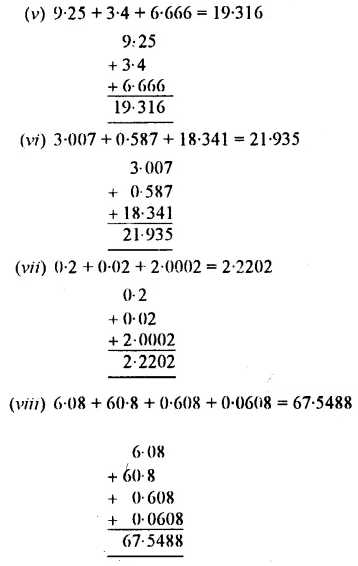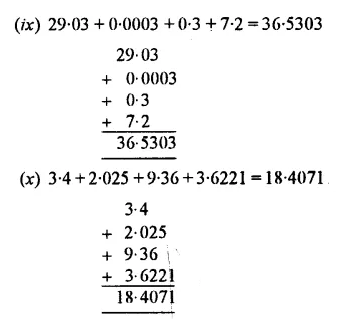Question 2.
Subtract the first! number from the second :
(i) 5.4, 9.8
(ii) 0.16, 4.3
(iii) 0.82, 8.6
(v) 2.237, 9.425
(vi) 41 .03, 59.46
(vii) 3.92. 26.86
(viii) 4.73, 8.5
(ix) 12.63, 36.2
(x) 0.845, 3.71
Solution:Question 3.
Simplify :
(i) 28.796 -13.42 – 2.555
(ii) 93.354 – 62.82 – 13.045
(iii) 36 – 18.59 – 3.2
(iv) 86 + 16.95 – 3.0042
(v) 32.8 – 13 – 10.725 +3.517
(vi) 4000 – 30.51 – 753.101 – 69.43
(vii) 0.1835 + 163.2005 – 25.9 – 100
(viii) 38.00 – 30 + 200.200 – 0.230
(ix) 555.555 + 55.555 – 5.55 – 0.555
Solution:Question 4.
Find the difference between 6.85 and 0.685.
Solution: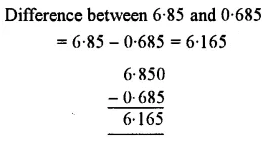Question 5.
Take out the sum of 19.38 and 56.025 then subtract it from 200. 111.
Solution:Question 6.
Add 13.95 and 1.003 ; and from the result, subtract the sum of 2.794 and 6.2.
Solution:Question 7.
What should be added to 39.587 to give 80.375 ?
Solution: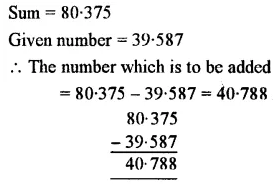Question 8.
What should be subtracted from 100 to give 19.29?
Solution: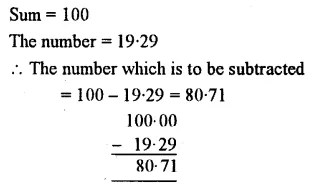Question 9.
What is the excess of 584.29 over 213.95 ?
Solution:Question 10.
Evaluate:
(i) (5.4 – 0.8) + (2.97 -1.462)
(ii) (6.25 + 0.36) -(17.2 – 8.97)
(iii) 9.004 + (3 -2.462)
(iv) 879.4 – (87.94 – 8 .794)
Solution: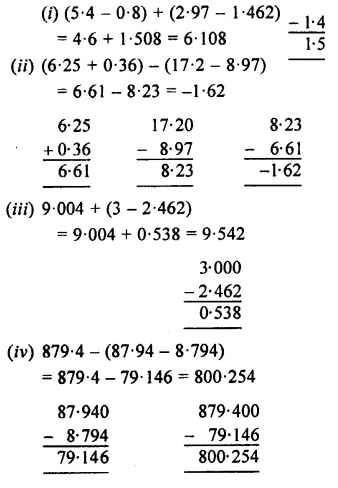Question 11.
What is the excess of 75 over 48.29?
Solution: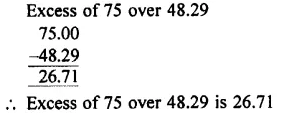Question 12.
If A = 237.98 and B = 83.47.
Find :
(i) A – B
(ii) B – A.
Solution: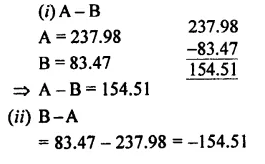Question 13.
The cost of one kg of sugar increases from ?28.47 to T32.65. Find the increase in cost.
Solution: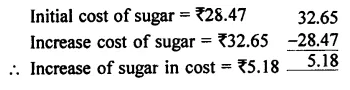### Decimal Fractions Exercise 4C – Selina Concise Mathematics Class 7 ICSE Solutions

Question 1.
Multiply:
(i) 0.87 by 10
(ii) 2.948 by 100
(iii) 6.4 by 1000
(iv) 5.8 by 4
(v) 16.32 by 28
(vi) 5. 037 by 8
(vi) 4.6 by 2.1
(viii) 0.568 by 6.4
Solution:Question 2.
Multiply each number by 10, 100, 1000 :
(i) 0.5
(ii) 0.112
(iii) 4.8
(iv) 0.0359
(v) 16.27
(vi) 234.8
Solution: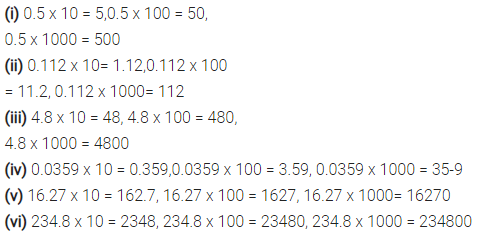Question 3.
Evaluate:
(i) 5.897 x 2.3
(ii) 0.894 x 87
(iii) 0.01 x 0.001
(iv) 0.84 x 2.2 x 4
(v) 4.75 x 0.08 x 3
(vi) 2.4 x 3.5 x 4.8
(vii) 0.8 x 1.2 x 0.25
(viii) 0.3 x 0.03 x 0.003
(ix) 12.003 x (0.2)5
Solution:Question 4.
Divide :
(i) 54.9 by 10
(ii) 7.8 by 100
(iii) 324.76 by 1000
(iv) 12.8 by 4
(v) 27.918 by 9
(vi) 4.672 by 8
(vii) 4.32 by 1.2
(viii) 7.644 by 1.4
(ix) 4.8432 by 0.08
Solution:Question 5.
Divide each of the given numbers by 10, 100, 1000 and 10000
(i) 2.1
(ii) 8.64
(iii) 5-01
(iv) 0.0906
(v) 0.125
(vi) 111.11
(vii) 0.848 x 3
(viii)4.906 x (0.2) ²
(ix) (1.2)² x(0.9)²
Solution: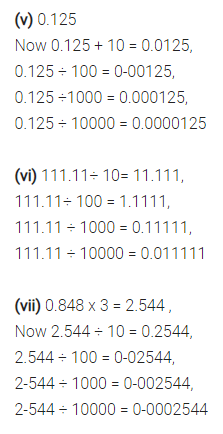Question 6.
Evaluate :
(i) 9.75 + 5
(ii) 4.4064 + 4
(iii) 27.69 + 30
(iv) 19.25 + 25
(v) 20.64+ 16
(vi) 3.204 + 9
(vii) 0.125 + 25
(viii) 0.14616 + 72
(ix) 0.6227+ 1300
(x) 257.894+ 0-169
(xi) 6.3 + (0.3)²
Solution: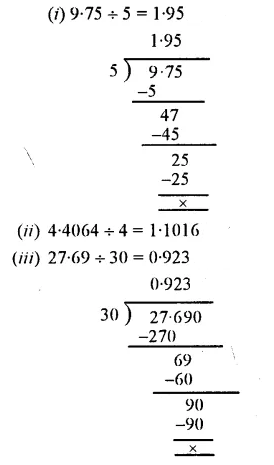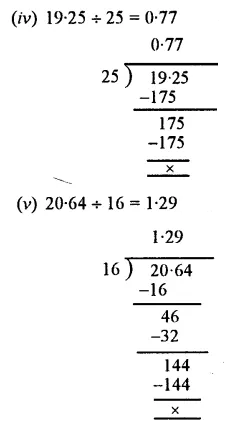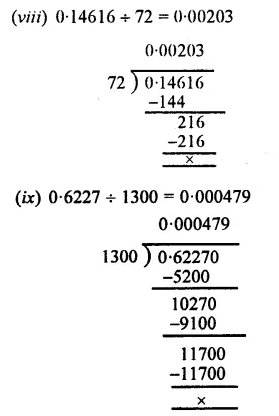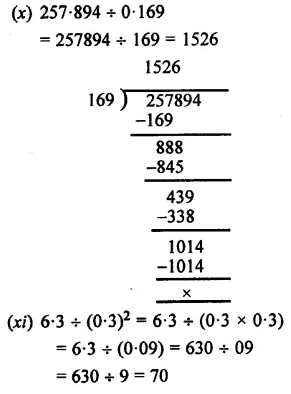Question 7.
Evaluate:
(i) 4.3 x 0.52 x 0.3
(ii) 3.2 x 2.5 x 0.7
(iii) 0.8 x 1.5 x 0.6
(iv) 0.3 x 0.3 x 0.3
(v) 1.2 x 1.2 x 0.4
(vi) 0.4 x 0.04 x 0.004
(vii) 0.5 x 0.6 x 0.7
(Viii) 0.5 x 0.06 x 0.007
Solution: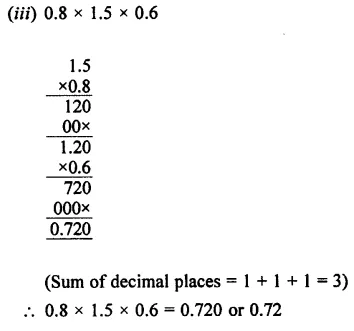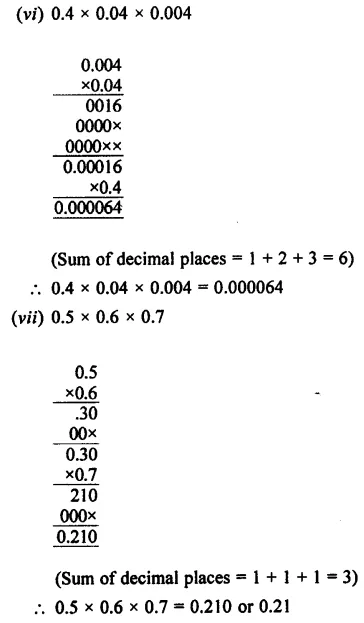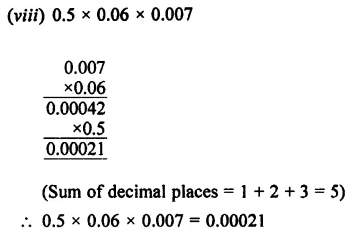Question 8.
Evaluate:
(i) (0.9)²
(ii) (0.6)² x 0.5
(iii) 0.3 x (0.5)²
(iv) (0.4)³
(v) (0.2)3 x 5
(vi) (0.2)3 x 0.05
Solution: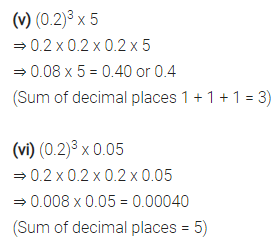Question 9.
Find the cost of 36.75 kg wheat at the rate of ₹12.80 per kg.
Solution: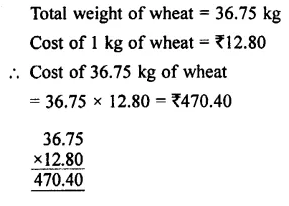Question 10.
The cost of a pen is ₹56.15. Find the cost of 16 such pens.
Solution: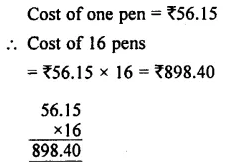Question 11.
Evaluate:
(i) 0.0072 ÷ 0.06
(ii) 0.621 ÷ 0.3
(iii) 0.0532 ÷ 0.005
(iv) 0.01162 ÷ 0.14
(v) (7.5 x 40.4) ÷ 25
(vi) 2.1 ÷ (0.1 x 0.1)
Solution:Question 12.
Fifteen indentical articles weigh 31.50 kg. Find the weight of each article.
Solution:Question 13.
The product of two numbers is 211.2. If one of these two numbers is 16.5, find the other number.
Solution: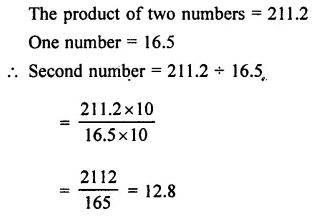Question 14.
One dozen identical articles cost ₹45.96. Find the cost of each article.
Solution: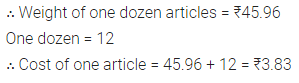### Decimal Fractions Exercise 4D – Selina Concise Mathematics Class 7 ICSE Solutions

Question 1.
Find whether the given division forms a terminating decimal or a non-terminating decimal:
(i) 3 ÷ 8
(ii)8 ÷ 3
(iii) 6÷ 5
(iv) 5 ÷ 6
(v) 12.5 ÷ 4
(vi) 23 ÷ 0.7
(vii) 42 ÷ 9
(viii) 0.56÷ 0.11
Solution: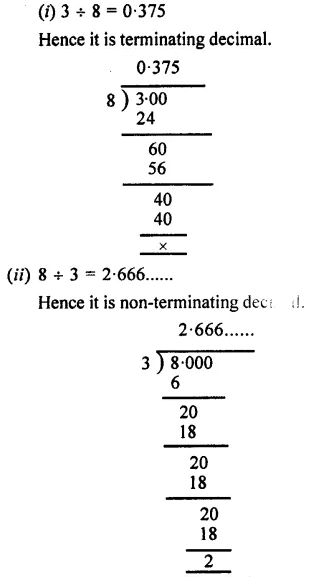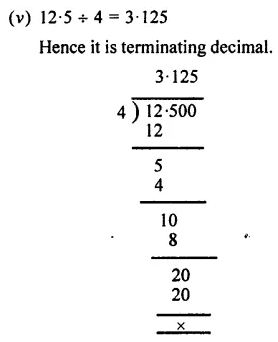Question 2.
Express as recurring decimals :Solution: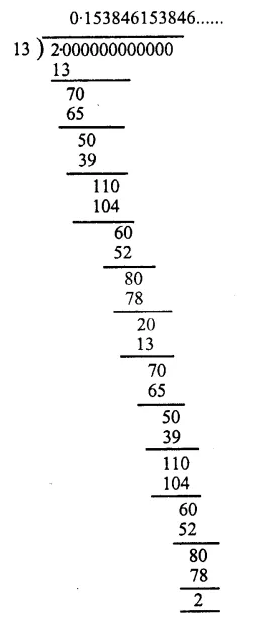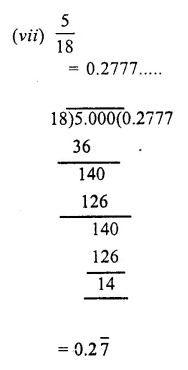Question 3.
Convert into vulgar fraction :
(i) 0.$$\bar { 3 }$$
(ii) 0.$$\bar { 8 }$$
(iii) 4.$$\bar { 4 }$$
(iv) 23.$$\bar { 7 }$$
Solution:Question 4.
Convert into vulgar fraction :
(i) 0.$$\bar { 35 }$$
(ii) 2.$$\bar { 23 }$$
(iii) 1.$$\bar { 28 }$$
(iv) 5.$$\bar { 234 }$$
Solution: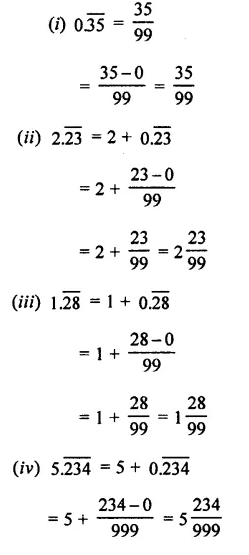Question 5.
Convert into vulgar fraction :
(i) 0 0.3$$\bar { 7 }$$
(ii) 0.2$$\bar { 45 }$$
(iii) 0.68$$\bar { 5 }$$
(iv) 0.4$$\bar { 42 }$$
Solution:### Decimal Fractions Exercise 4E – Selina Concise Mathematics Class 7 ICSE Solutions

Question 1.
Round off:
(i) 0 .07, 0.112, 3.59, 9.489 to the nearest tenths.
(ii) 0.627, 100.479, 0 065 and 0.024 to the nearest hundredths.
(iii) 4.83,0.86,451 .943 and 9.08 to the nearest whole number.
Solution:Question 2.
(i) 18 .35 x 1.2
(ii) 62.89 x 0.02
Solution:Question 3.
Write the number of significant figures (digits) in:
(i) 35.06
(ii) 0.35
(iii) 7.0068
(iv) 19 .0
(v) 0.0062
(vi) 0 4.2 x 0.6
(vii) 0.08 x 25
(viii) 3.6 ÷ 0.12 .
Solution:Question 4.
Write :
(i) 35.869,0 008426,4.952 and 382.7, correct lo three significant figures.
(ii) 60.974. 2.8753, 0.001789 and 400.04, correct to four significant figures.
(iii) 14.29462, 19.2, 46356.82 and 69, correct to five significant figures.
Solution:### Decimal Fractions Exercise 4F – Selina Concise Mathematics Class 7 ICSE Solutions

Question 1.
The weight of an object is 3 .06 kg. Find the total weight of 48 similar objects.
Solution:Question 2.
Find die cost of 17.5 m cloth at the rate of Rs. 112.50 per metre.
Solution: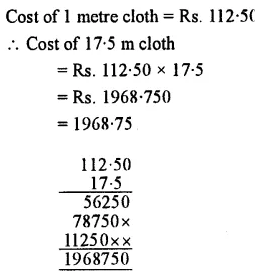Question 3.
One kilogramme of oil costs Rs. 73.40. Find the cost of 9.75 kilogramme of the oil.
Solution:Question 4.
Total weight of 8 identical objects is 51.2 kg. Find the weight of each object.
Solution: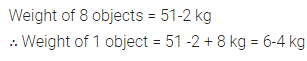Question 5.
18.5 m of cloth costs Rs. 666. Find the cost of 3.8 m cloth.
Solution:Question 6.
Find die value of:
(i) 0.5 of Rs. 7.60 + 1.62 of Rs. 30
(ii) 2.3 of 7.3 kg + 0.9 of 0.48 kg
(iii) 6.25 of 8.4 – 4.7 of 3.24
(iv) 0.98 of 235 – 0 .09 of 3.2
Solution:Question 7.
Evaluate:
(i) 5.6 – 1 .5 of 3.4
(ii) 4.8 ÷ 0.04 of 5
(iii) 0.72 of 80 + 0.2
(iv) 0.72 ÷ 80 of 0.2
(v) 6.45 + (3.9 – 1.75)
(vi) 0.12 of(0.104 – 0.02)+ 0.36 x 0.5
Solution: